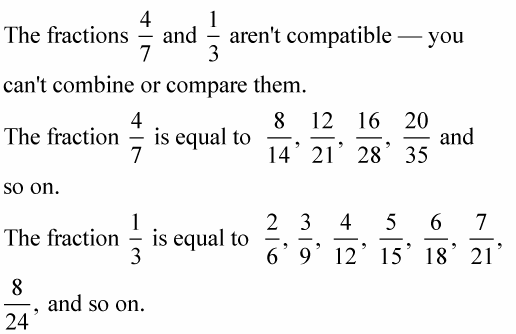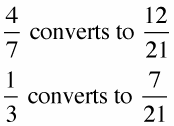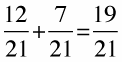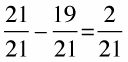You can subtract proper fractions that have different denominators — such as thirds and sixths — if you find the common denominator first. The biggest step in subtracting proper fractions is finding the common denominator, but once you've done that, you can subtract the numerators just like any other basic subtraction problem.

Example: In her will, Jane gave 4/7 of her money to the Humane Society and 1/3 of her money to other charities. How much was left for her children’s inheritance?

1. Convert the fractions so that they have the same number in the denominators.Can you spot the common denominator among all those fractions? Both fractions have a common denominator, 21. So now,2. Add or subtract the numerators, and leave the denominators alone.

Add the numerators to get the total designation to charity in Jane's will:Subtract that result from the whole of Jane’s money (21/21) to determine the portion allotted to her children:3. Reduce the answer, if needed.

In this case, 2/21 is the answer — the amount of Jane's estate that Jane's children will be awarded — and the answer doesn't need to be reduced.